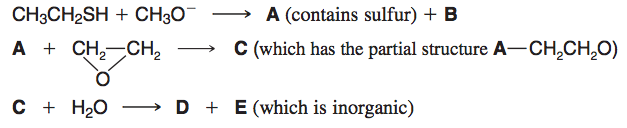# Problem: (a) Given the above sequence of reactions, draw structures for A through E.

###### Problem Details

(a) Given the above sequence of reactions, draw structures for A through E.What scientific concept do you need to know in order to solve this problem?

Our tutors have indicated that to solve this problem you will need to apply the Epoxide Reactions concept. You can view video lessons to learn Epoxide Reactions. Or if you need more Epoxide Reactions practice, you can also practice Epoxide Reactions practice problems.

What is the difficulty of this problem?

Our tutors rated the difficulty of(a) Given the above sequence of reactions, draw structures f...as low difficulty.

How long does this problem take to solve?

Our expert Organic tutor, Jonathan took 3 minutes and 25 seconds to solve this problem. You can follow their steps in the video explanation above.

What textbook is this problem found in?

Our data indicates that this problem or a close variation was asked in Organic Chemistry - Solomons 10th Edition. You can also practice Organic Chemistry - Solomons 10th Edition practice problems.